# DAV Class 8 Maths Chapter 15 Brain Teasers Solutions

The DAV Class 8 Maths Solution and DAV Class 8 Maths Chapter 15 Brain Teasers Solutions of Statistics and Probability offer comprehensive answers to textbook questions.

## DAV Class 8 Maths Ch 15 Brain Teasers Solutions

A1. Tick (✓) the correct option.

(i) A survey of 100 people found that 20 of them played badminton. In a pie chart, what would be the sector angle of this group?
(a) 20°
(b) 36°
(c) 72°
(d) 200°
Solution:
(c) 72°
Number of total people participating in survey = 100
Number of people playing badminton = 20
Fraction of people playing badminton = $$\frac{20}{100}=\frac{1}{5}$$
The sector angle of this group in pie chart = 360° × $$\frac{1}{5}$$ = 72°
Thus, the option (c) is the correct answer.

(ii) Representing data graphically in the form of rectangles, with no gap between the successive rectangles is called
(a) bar graph
(b) histograph
(c) pie chart
(d) histogram
Solution:
(d) histogram
Histogram
The option (d) is the correct answer.(iii) What is the probability of getting a king, if a card is drawn out from a pack of 52 cards?
(a) $$\frac{1}{52}$$
(b) $$\frac{2}{52}$$
(c) $$\frac{3}{52}$$
(d) $$\frac{4}{52}$$
Solution:
Number of total cards = 52
Number of kings = 4
Probability of drawing kings = $$\frac{4}{52}$$
Thus, option (d) is the correct answer.

(iv) A histogram has
(a) frequencies as heights
(b) class intervals as heights
(c) frequencies as bases
(d) frequencies as both bases and heights
Solution:
(a) frequencies as heights
In a histogram, heights of rectangles represent frequencies.
Thus, option (a) is the correct answer.

(v) The possible number of outcomes if the coin is tossed twice are
(a) one
(b) two
(c) three
(d) four
Solution:
(d) four
A coin has two faces – Head and Tail.
Thus, there are four possible number of outcomes if the coin is tossed twice.
Option (d) is the correct answer.(i) A letter is chosen from the word ‘PROBABILITY’. What is the probability that it is a vowel?
Solution:
In the given word : PROBABILITY,
Number of vowels = 4
Total number of letters = 11
Probability of vowels = $$\frac{4}{11}$$

(ii) Heights of 20 saplings in a nursery is noted as (in cm):
80, 79, 102, 90, 94, 124, 111, 87, 88, 103, 72, 126, 125, 88, 98, 96, 127, 128, 74, 80
Solution:
Maximum height of the sapling = 128 cm
Minimum height of the sapling = 72 cm
Range of heights = 128 – 72 = 56 cm

(iii) A number is selected at random from the first ten natural numbers. What will be the probability of the number being prime?
Solution:
First ten natural numbers are 1, 2, 3, 4, 5, 6, 7, 8, 9, 10.
Prime numbers are 2, 3, 5, 7.
Number of prime numbers = 4
Probability of prime numbers = $$\frac{4}{10}$$(iv) A survey was conducted to find out the type of story books read by a group of 180 children. The data was represented as a pie chart given below.Solution:
Part of circle representing adventure books = $$\frac{20}{100}=\frac{1}{5}$$
Total number of children = 180
Number of children who read adventure books = 180 × $$\frac{1}{5}$$ = 36

(v) From the above pie chart, what fraction of children do not read story books at all?
Solution:
Part of circle representing the number of children who do not read story books = $$\frac{10}{100}=\frac{1}{10}$$
Number of children who do not read story books = 180 × $$\frac{1}{10}$$ = 18
Fraction of children who do not read story books = $$\frac{18}{180}=\frac{1}{10}$$Question 2.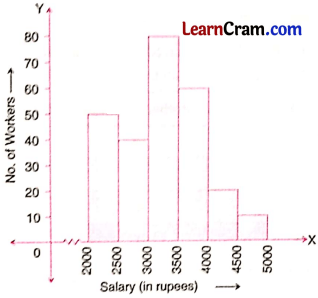(i) What information is depicted by the above histogram?
Solution:
The given histogram depicts the information of salary of workers.

(ii) Prepare a grouped frequency table for the histogram.
Solution: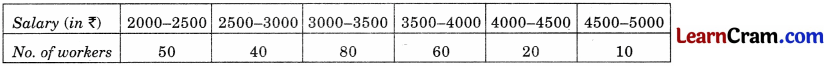(iii) Find the number of workers having salaries more than ₹ 3,500.
Solution:
90 workers are having their salary more than ₹ 3500.

(iv) In which group is the number of workers maximum?
Solution:
3000 – 3500

(v) In which group is the number of workers minimum?
Solution:
4500 – 5000

(vi) What are the class marks of all the class intervals?
Solution:
Class marks are 2250, 2750, 3250, 3750, 4250 and 4750 respectively.Question 3.
The water bill (in ₹) of 30 houses in a locality are given below. Construct a grouped frequency distribution with class size 15.
30 33 45 54 74 78 108 112 66 76 88 40 14 20 15
35 44 66 75 84 95 96 102 110 88 74 112 14 34 44.
Solution: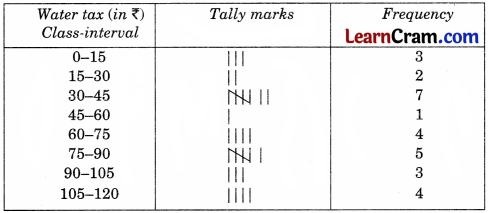Question 4.
The following is the distribution of weights (in kg) of 50 persons:Draw a histogram for the above data.
Solution: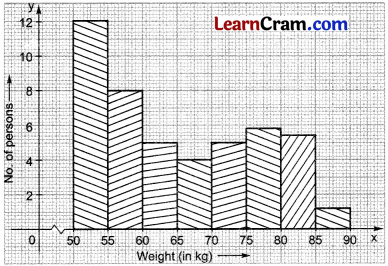Question 5.
The given pie chart represents the amount spent on different sports by a school administration in a year. If the total money spent on football is ₹ 5000, answer the following questions.
(i) What is the amount spent on sports?
(ii) How much money is spent on hockey?
(iii) How much more money is spent on cricket than hockey?
Solution:
(i) Money spent of football = ₹ 5000
∴ Total money spent on sports = $$\frac{360}{50}$$ × 5000 = ₹ 36000
(ii) Money spent on hockey = $$\frac{100}{360}$$ × 36000 = ₹ 10000
(iii) Money spent on cricket = $$\frac{100}{360}$$ × 36000 = ₹ 16000
Money spent more on cricket than hockey = ₹ 16000 – ₹ 10000 = ₹ 6000

Question 6.
The following table shows the colours preferred by a group of people. Draw a pie chart showing the information.

 Colours No. of people Blue 18 Green 9 Red 6 Yellow 3

Solution: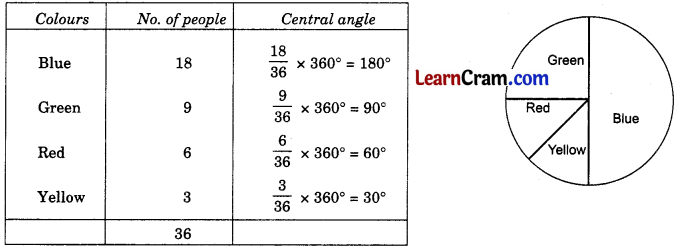Question 7.
When a die is thrown, list the outcomes of an event of getting
(i) a prime number
(ii) a composite number
(iii) a number less than 4
(iv) a number more than or equal to 3.
Solution:
(i) {2, 3, 5} are the prime numbers
(ii) {4, 6} are the composite numbers
(iii) {1, 2, 3} are the numbers less than 4
(iv) {3, 4, 5, 6} are the numbers more or equal to 3.

Question 8.
The following numbers were written in separate slips and mixed in a bag. You are asked to pick a slip. What is the probability of getting:
(i) an even number?
(ii) a multiple of 3?
(iii) a multiple of 3 but not 9?
(iv) a factor of 36?
(v) a one-digit number?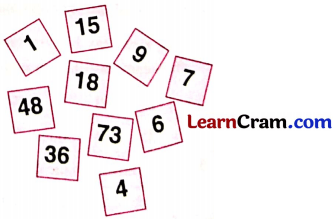Solution:
Total number of slips = 10
(i) Probability of getting even numbers = $$\frac{5}{10}=\frac{1}{2}$$
(ii) Probability of getting a multiple of 3 = $$\frac{6}{10}=\frac{3}{5}$$
(iii) Probability of getting a multiple of 3 but not 9 = $$\frac{3}{10}$$
(iv) Probability of getting a factor of 36 = $$\frac{4}{10}=\frac{2}{5}$$
(v) Probability of getting a one-digit number = $$\frac{5}{10}=\frac{1}{2}$$.### DAV Class 8 Maths Chapter 15 HOTS

Question 1.
The given pie chart shows the games played by 1,500 students of a school. Each student plays only one game. Find the ratio of the students who play hockey to those who play badminton.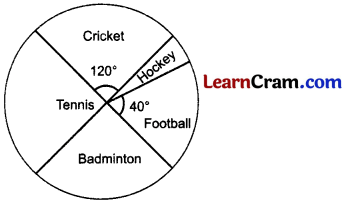Solution:
Since sector angles for cricket and badminton are vertically opposite angles.
∴ Sector angle for Cricket = Sector angle for Badminton = 120°.
Let the sector angle for hockey be x.
Here, sector angles for cricket, hockey and football form a straight angle.
∴ 120° + x + 40° = 180° x + 160° = 180°
⇒ x = 20°
Total number of students playing games = 1500 (Given)
Part of circle representing hockey = $$\frac{20^{\circ}}{360^{\circ}}=\frac{1}{18}$$
Number of students who play hockey = 1500 × $$\frac{1}{18}$$ = $$\frac{250}{3}$$
Part of circle representing badminton = $$\frac{120^{\circ}}{360^{\circ}}=\frac{1}{3}$$
Number of students who play badminton = 1500 × $$\frac{1}{3}$$ = 500
Ratio of the students who play hockey to those who play badminton = $$\frac{250}{\frac{3}{500}}$$
= $$\frac{250}{3 \times 500}$$
= $$\frac{1}{6}$$ = 1 : 6.Question 1.
Construct a histogram for the following distribution:

 Daily earning (in ₹) 80 – 120 120 – 160 160 – 200 200 – 240 No. of workers 4 8 10 2

Solution: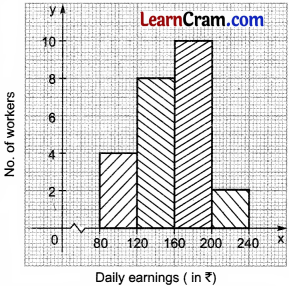Question 2.
Prepare a frequency distribution table from the given data.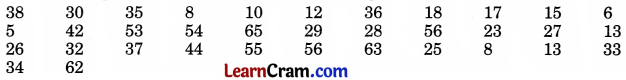Solution: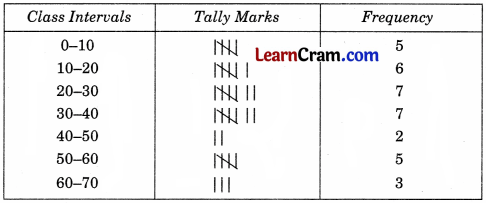Question 3.
Marks obtained by 40 students in an examination are given below. Draw histogram.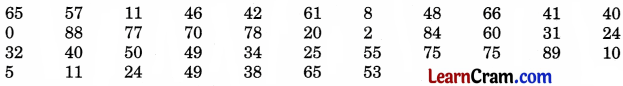Solution:Question 4.
The following table represent the games and the number of students taking part in their interested games. Draw a pie chart for the given data:

 Games No. of students Hockey 36 Cricket 18 Badminton 27 Football 12 Khokho 15

Solution: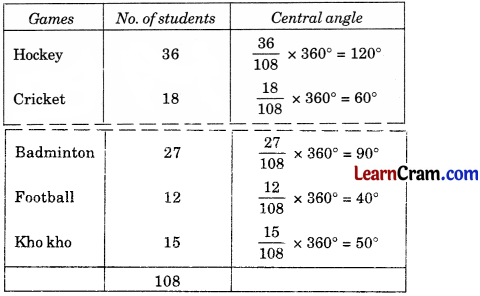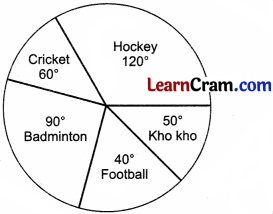Question 5.
A box contains 24 marbles of different colours. The following table shows number of marbles of different colours. Draw a pie chart for the data.

 Colour of marbles Red Green Yellow Blue No. of marbles 10 6 5 3

Solution: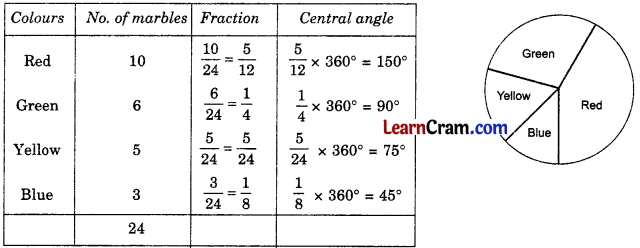Question 6.
Two cards are randomly drawn from a deck of 52 playing cards. Find the probability that
(i) both are aces of red colour
(ii) both are kings of black colour.
Solution:
Here n(s) = 52
(i) Number of red colour aces = 2
∴ n(E) = 2
∴ Probability of getting 2 aces of red colour = $$\frac{n(\mathrm{E})}{n(\mathrm{~S})}$$
= $$\frac{2}{52}=\frac{1}{26}$$

(ii) Number of black kings = 2
∴ n(E) = 2
∴ Probability of getting 2 black kings = $$\frac{n(\mathrm{E})}{n(\mathrm{~S})}$$
= $$\frac{2}{52}=\frac{1}{26}$$Question 7.
A coin is tossed 3 times. Find the probability of getting
(ii) 3 tails
(iv) atleast 2 tails
Solution:
Total number of outcomes = 8
Sample space are : [HHH, HHT, HTH, HTT, THH, THT, TTH, TTT]

(i) P(3 heads) = $$\frac{1}{8}$$
(ii) P(3 tails) = $$\frac{1}{8}$$
(iii) P(atleast 1 head) = $$\frac{7}{8}$$
(iv) P(atleast 2 tails) = $$\frac{4}{8}=\frac{1}{2}$$

Question 8.
Among the quantity of fruits sold in one day, sale of mangoes is 50%. The angle of sector representing mangoes in the pie-chart.
(a) 50°
(b) 90°
(c) 360°
(d) 180°
Solution:
(d) 180°
Sector angle = $$\frac{50}{100}$$ × 360° = 180°
So, (d) is correct.Question 9.
A bag contains 5 red balls, 8 white balls, 4 green balls and 7 black balls. If one ball is drawn at random, find the probability that it is
(a) black ball
(b) red ball
(c) not a green ball
Solution:
Total number of balls in the bag = 5 red + 8 white + 4 green + 7 black = 24 balls
(a) Probability of getting a black ball = $$\frac{7}{24}$$
(b) Probability of getting a red ball = $$\frac{5}{24}$$
(c) Probability of getting no green ball = $$\frac{20}{24}=\frac{5}{6}$$

Question 10.
The pulse rate (per minute) of 30 persons is recorded asConstruct a frequency table using class-intervals 60-65, 65-70 etc.
(i) What is the upper limit of third class interval?
(ii) What are the class marks of each class interval?
(iii) Which class interval has the highest frequency?
Solution:(i) Upper limit of third class interval is 75.
(ii) Class marks of each interval are: 62.5, 67.5, 71.5, 77.5, 82.5
(iii) 70-75 has the highest frequency 14.Question 11.
A card is drawn at random from a pack of 52 cards. Find the probability that the card drawn is
(i) a black king
(ii) a red card
(iii) neither a red card nor a queen
(iv) a heart
(v) other than an ace
(vi) a Jack.
Solution:
Total number of sample space = 52

(i) Probability of getting a black king = $$\frac{2}{52}=\frac{1}{26}$$

(ii) Probability of getting a red card = $$\frac{26}{52}=\frac{1}{2}$$

(iii) Probability of getting neither a red card nor a queen = $$\frac{30}{52}=\frac{15}{26}$$

(iv) Probability of getting a heart = $$\frac{13}{52}=\frac{1}{4}$$

(v) Probability of getting a card other than ace = $$\frac{48}{52}=\frac{12}{13}$$

(vi) Probability of getting a Jack = $$\frac{4}{52}=\frac{1}{13}$$Question 12.
The electricity bill (in ₹) of 25 houses of a certain locality for a month are given below:Prepare a frequency table taking class intervals 300 – 400, 400 – 500 etc. Also draw a histogram.
Solution: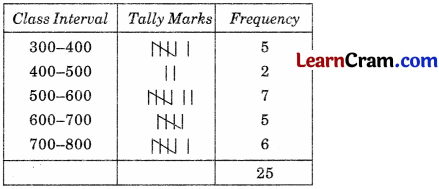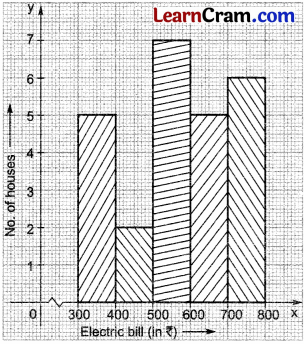Question 13.
A box contains 24 marbles of different colours. The following table shows number of marbles of these thiferent colours. Draw a pie chart for the data.

 Colour of marble Red Green Yellow Blue No. of marbles 10 6 5 3

Solution: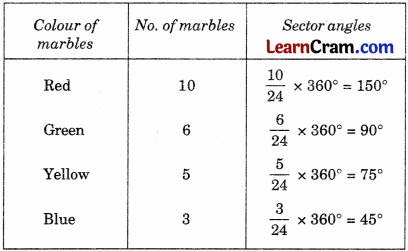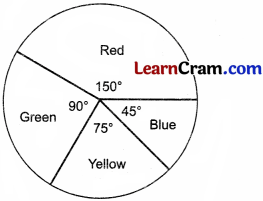Multiple Choice Questions:

Question 1.
If a fair coin is tossed four times then the number of outcomes is
(a) 12
(b) 8
(c) 16
(d) 32
Solution:
(c) 16

Question 2.
If a fair die is rolled once then the probability of getting a prime number is
(a) $$\frac{1}{2}$$
(b) $$\frac{1}{3}$$
(c) $$\frac{1}{4}$$
(d) none of these.
Solution:
(a) $$\frac{1}{2}$$

Question 3.
A fair die is rolled, the probability of getting a number greater than 6 is
(a) 1
(b) 0
(c) $$\frac{1}{2}$$
(d) $$\frac{1}{6}$$
Solution:
(b) 0Question 4.
A card is drawn randomly from a well-shuffled pack of playing cards. The probability of getting an ace is
(a) $$\frac{1}{4}$$
(b) $$\frac{1}{13}$$
(c) $$\frac{1}{26}$$
(d) $$\frac{1}{16}$$
Solution:
(b) $$\frac{1}{13}$$

Question 5.
Ten slips are made with different digits from 0 to 9, the probability of getting a slip with odd digits is
(a) $$\frac{1}{3}$$
(b) $$\frac{1}{2}$$
(c) $$\frac{1}{5}$$
(d) $$\frac{1}{4}$$
Solution:
(b) $$\frac{1}{2}$$